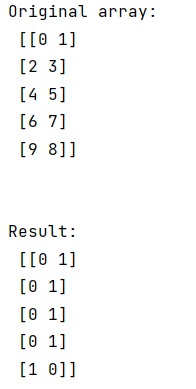# How to change max in each row to 1, all other numbers to 0 in NumPy array?

Given a NumPy array, we have to change max in each row to 1, all other numbers to 0 in it.
Submitted by Pranit Sharma, on March 12, 2023

## Changing max in each row to 1, all other numbers to 0

Suppose that we are given a multidimensional NumPy array and we need to implement a function so that it sets the max values of each row of the array as 1, and the rest all the values as 0.

To change the max in each row to 1 and all other numbers to 0, we will first filter out all the max values from each row and also we will convert the shape of the result. We will then use numpy.where() and pass a condition that if array is equal to the result of max values result, it will set the array values as 1 at that point otherwise 0.

Let us understand with the help of an example,

## Python code to change max in each row to 1, all other numbers to 0 in NumPy array

```# Import numpy
import numpy as np

# Creating a numpy array
arr = np.array([[0, 1],[2, 3],[4, 5],[6, 7],[9, 8]])

# Display Original array
print("Original array:\n",arr,"\n\n")

# Getting max values by row and changing dimension
max = arr.max(axis=1).reshape(-1, 1)

# Setting max values as 1 and other as 0
res = np.where(arr == max, 1, 0)

# Display result
print("Result:\n",res)
```

### OutputLanguages: » C » C++ » C++ STL » Java » Data Structure » C#.Net » Android » Kotlin » SQL
Web Technologies: » PHP » Python » JavaScript » CSS » Ajax » Node.js » Web programming/HTML
Solved programs: » C » C++ » DS » Java » C#
Aptitude que. & ans.: » C » C++ » Java » DBMS
Interview que. & ans.: » C » Embedded C » Java » SEO » HR
CS Subjects: » CS Basics » O.S. » Networks » DBMS » Embedded Systems » Cloud Computing
» Machine learning » CS Organizations » Linux » DOS
More: » Articles » Puzzles » News/Updates